Anzeige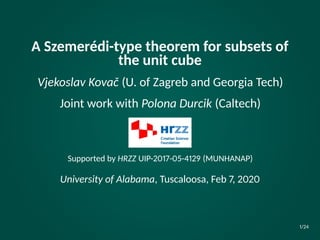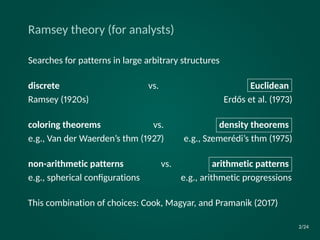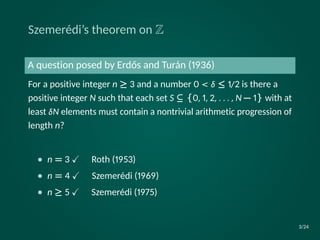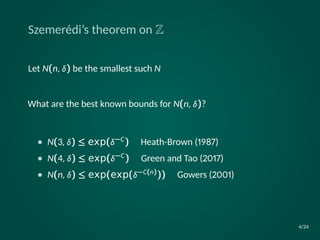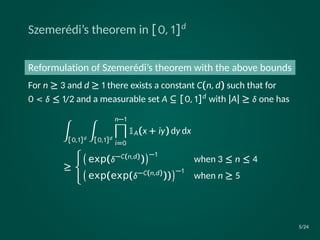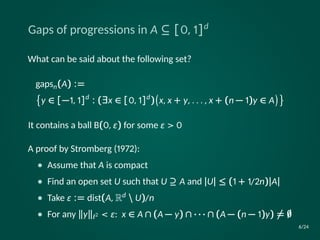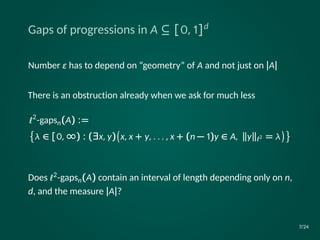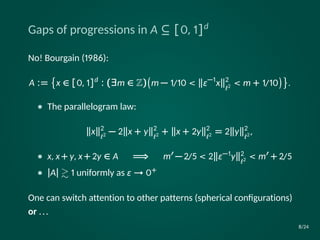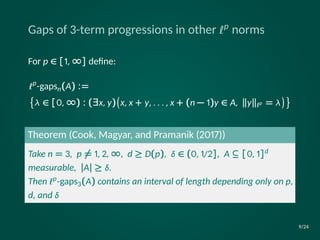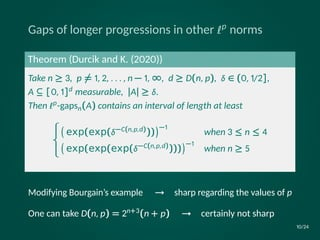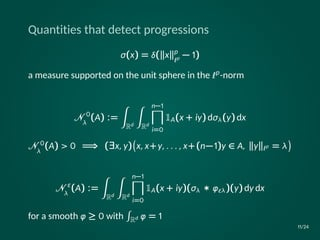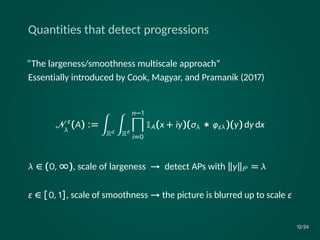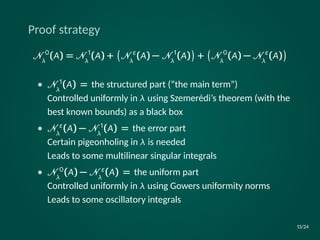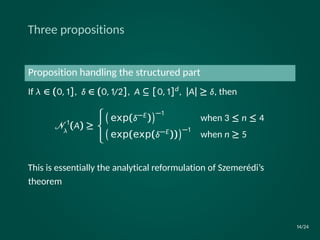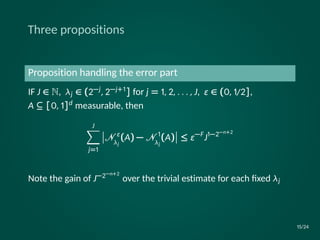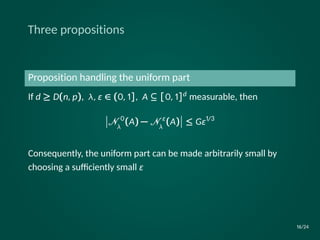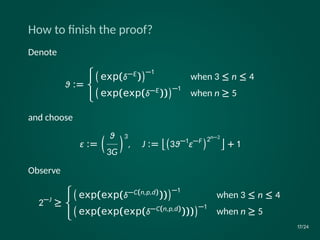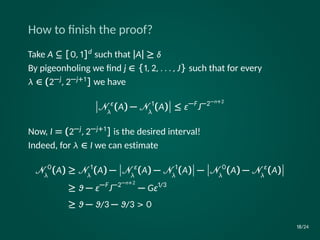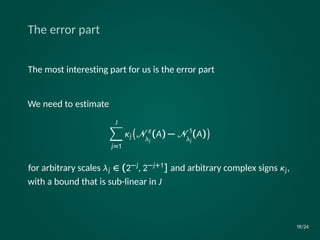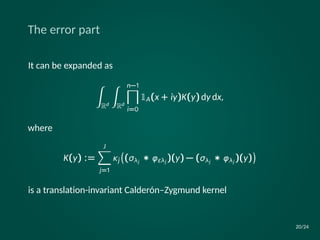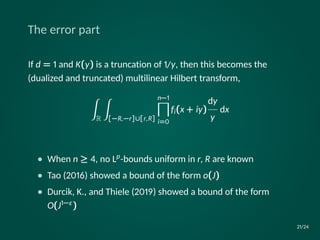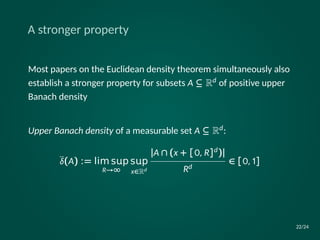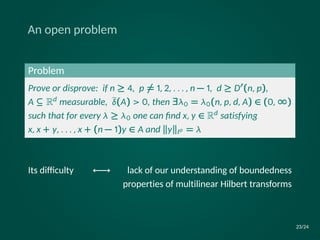1 von 24
Anzeige

### A Szemerédi-type theorem for subsets of the unit cube

1. A Szemerédi-type theorem for subsets of the unit cube Vjekoslav Kovač (U. of Zagreb and Georgia Tech) Joint work with Polona Durcik (Caltech) Supported by HRZZ UIP-2017-05-4129 (MUNHANAP) University of Alabama, Tuscaloosa, Feb 7, 2020 1/24
2. Ramsey theory (for analysts) Searches for patterns in large arbitrary structures discrete vs. Euclidean Ramsey (1920s) Erdős et al. (1973) coloring theorems vs. density theorems e.g., Van der Waerden’s thm (1927) e.g., Szemerédi’s thm (1975) non-arithmetic patterns vs. arithmetic patterns e.g., spherical conﬁgurations e.g., arithmetic progressions This combination of choices: Cook, Magyar, and Pramanik (2017) 2/24
3. Szemerédi’s theorem on Z A question posed by Erdős and Turán (1936) For a positive integer n ≥ 3 and a number 0 < δ ≤ 1/2 is there a positive integer N such that each set S ⊆ {0, 1, 2, . . . , N − 1} with at least δN elements must contain a nontrivial arithmetic progression of length n? • n = 3 Roth (1953) • n = 4 Szemerédi (1969) • n ≥ 5 Szemerédi (1975) 3/24
4. Szemerédi’s theorem on Z Let N(n, δ) be the smallest such N What are the best known bounds for N(n, δ)? • N(3, δ) ≤ exp(δ−C) Heath-Brown (1987) • N(4, δ) ≤ exp(δ−C) Green and Tao (2017) • N(n, δ) ≤ exp(exp(δ−C(n))) Gowers (2001) 4/24
5. Szemerédi’s theorem in [0, 1]d Reformulation of Szemerédi’s theorem with the above bounds For n ≥ 3 and d ≥ 1 there exists a constant C(n, d) such that for 0 < δ ≤ 1/2 and a measurable set A ⊆ [0, 1]d with |A| ≥ δ one has ∫︁ [0,1]d ∫︁ [0,1]d n−1∏︁ i=0 1A(x + iy) dy dx ≥ ⎧ ⎨ ⎩ (︀ exp(δ−C(n,d)) )︀−1 when 3 ≤ n ≤ 4 (︀ exp(exp(δ−C(n,d))) )︀−1 when n ≥ 5 5/24
6. Gaps of progressions in A ⊆ [0, 1]d What can be said about the following set? gapsn(A) := {︀ y ∈ [−1, 1]d : (∃x ∈ [0, 1]d ) (︀ x, x + y, . . . , x + (n − 1)y ∈ A )︀}︀ It contains a ball B(0, ϵ) for some ϵ > 0 A proof by Stromberg (1972): • Assume that A is compact • Find an open set U such that U ⊇ A and |U| ≤ (1 + 1/2n)|A| • Take ϵ := dist(A, Rd U)/n • For any y ℓ2 < ϵ: x ∈ A ∩ (A − y) ∩ · · · ∩ (A − (n − 1)y) = 6/24
7. Gaps of progressions in A ⊆ [0, 1]d Number ϵ has to depend on “geometry” of A and not just on |A| There is an obstruction already when we ask for much less ℓ2 -gapsn(A) := {︀ λ ∈ [0, ∞) : (∃x, y) (︀ x, x + y, . . . , x + (n − 1)y ∈ A, y ℓ2 = λ )︀}︀ Does ℓ2-gapsn(A) contain an interval of length depending only on n, d, and the measure |A|? 7/24
8. Gaps of progressions in A ⊆ [0, 1]d No! Bourgain (1986): A := {︀ x ∈ [0, 1]d : (∃m ∈ Z) (︀ m − 1/10 < ϵ−1 x 2 ℓ2 < m + 1/10 )︀}︀ . • The parallelogram law: x 2 ℓ2 − 2 x + y 2 ℓ2 + x + 2y 2 ℓ2 = 2 y 2 ℓ2 , • x, x+ y, x+ 2y ∈ A =⇒ m − 2/5 < 2 ϵ−1y 2 ℓ2 < m + 2/5 • |A| 1 uniformly as ϵ → 0+ One can switch attention to other patterns (spherical conﬁgurations) or ... 8/24
9. Gaps of 3-term progressions in other ℓp norms For p ∈ [1, ∞] deﬁne: ℓp -gapsn(A) := {︀ λ ∈ [0, ∞) : (∃x, y) (︀ x, x + y, . . . , x + (n − 1)y ∈ A, y ℓp = λ )︀}︀ Theorem (Cook, Magyar, and Pramanik (2017)) Take n = 3, p = 1, 2, ∞, d ≥ D(p), δ ∈ (0, 1/2], A ⊆ [0, 1]d measurable, |A| ≥ δ. Then ℓp-gaps3(A) contains an interval of length depending only on p, d, and δ 9/24
10. Gaps of longer progressions in other ℓp norms Theorem (Durcik and K. (2020)) Take n ≥ 3, p = 1, 2, . . . , n − 1, ∞, d ≥ D(n, p), δ ∈ (0, 1/2], A ⊆ [0, 1]d measurable, |A| ≥ δ. Then ℓp-gapsn(A) contains an interval of length at least ⎧ ⎨ ⎩ (︀ exp(exp(δ−C(n,p,d))) )︀−1 when 3 ≤ n ≤ 4 (︀ exp(exp(exp(δ−C(n,p,d)))) )︀−1 when n ≥ 5 Modifying Bourgain’s example → sharp regarding the values of p One can take D(n, p) = 2n+3(n + p) → certainly not sharp 10/24
11. Quantities that detect progressions σ(x) = δ( x p ℓp − 1) a measure supported on the unit sphere in the ℓp-norm 0 λ (A) := ∫︁ Rd ∫︁ Rd n−1∏︁ i=0 1A(x + iy) dσλ(y) dx 0 λ (A) > 0 =⇒ (∃x, y) (︀ x, x+y, . . . , x+(n−1)y ∈ A, y ℓp = λ )︀ ϵ λ (A) := ∫︁ Rd ∫︁ Rd n−1∏︁ i=0 1A(x + iy)(σλ ∗ φϵλ)(y) dy dx for a smooth φ ≥ 0 with ∫︀ Rd φ = 1 11/24
12. Quantities that detect progressions “The largeness/smoothness multiscale approach” Essentially introduced by Cook, Magyar, and Pramanik (2017) ϵ λ (A) := ∫︁ Rd ∫︁ Rd n−1∏︁ i=0 1A(x + iy)(σλ ∗ φϵλ)(y) dy dx λ ∈ (0, ∞), scale of largeness → detect APs with y ℓp = λ ϵ ∈ [0, 1], scale of smoothness → the picture is blurred up to scale ϵ 12/24
13. Proof strategy 0 λ (A) = 1 λ (A) + (︀ ϵ λ (A) − 1 λ (A) )︀ + (︀ 0 λ (A) − ϵ λ (A) )︀ • 1 λ (A) = the structured part (“the main term”) Controlled uniformly in λ using Szemerédi’s theorem (with the best known bounds) as a black box • ϵ λ (A) − 1 λ (A) = the error part Certain pigeonholing in λ is needed Leads to some multilinear singular integrals • 0 λ (A) − ϵ λ (A) = the uniform part Controlled uniformly in λ using Gowers uniformity norms Leads to some oscillatory integrals 13/24
14. Three propositions Proposition handling the structured part If λ ∈ (0, 1], δ ∈ (0, 1/2], A ⊆ [0, 1]d, |A| ≥ δ, then 1 λ (A) ≥ ⎧ ⎨ ⎩ (︀ exp(δ−E) )︀−1 when 3 ≤ n ≤ 4 (︀ exp(exp(δ−E)) )︀−1 when n ≥ 5 This is essentially the analytical reformulation of Szemerédi’s theorem 14/24
15. Three propositions Proposition handling the error part IF J ∈ N, λj ∈ (2−j, 2−j+1] for j = 1, 2, . . . , J, ϵ ∈ (0, 1/2], A ⊆ [0, 1]d measurable, then J∑︁ j=1 ⃒ ⃒ ϵ λj (A) − 1 λj (A) ⃒ ⃒ ≤ ϵ−F J1−2−n+2 Note the gain of J−2−n+2 over the trivial estimate for each ﬁxed λj 15/24
16. Three propositions Proposition handling the uniform part If d ≥ D(n, p), λ, ϵ ∈ (0, 1], A ⊆ [0, 1]d measurable, then ⃒ ⃒ 0 λ (A) − ϵ λ (A) ⃒ ⃒ ≤ Gϵ1/3 Consequently, the uniform part can be made arbitrarily small by choosing a suﬃciently small ϵ 16/24
17. How to ﬁnish the proof? Denote ϑ := ⎧ ⎨ ⎩ (︀ exp(δ−E) )︀−1 when 3 ≤ n ≤ 4 (︀ exp(exp(δ−E)) )︀−1 when n ≥ 5 and choose ϵ := (︁ ϑ 3G )︁3 , J := ⌊︀(︀ 3ϑ−1 ϵ−F )︀2n−2 ⌋︀ + 1 Observe 2−J ≥ ⎧ ⎨ ⎩ (︀ exp(exp(δ−C(n,p,d))) )︀−1 when 3 ≤ n ≤ 4 (︀ exp(exp(exp(δ−C(n,p,d)))) )︀−1 when n ≥ 5 17/24
18. How to ﬁnish the proof? Take A ⊆ [0, 1]d such that |A| ≥ δ By pigeonholing we ﬁnd j ∈ {1, 2, . . . , J} such that for every λ ∈ (2−j, 2−j+1] we have ⃒ ⃒ ϵ λ (A) − 1 λ (A) ⃒ ⃒ ≤ ϵ−F J−2−n+2 Now, I = (2−j, 2−j+1] is the desired interval! Indeed, for λ ∈ I we can estimate 0 λ (A) ≥ 1 λ (A) − ⃒ ⃒ ϵ λ (A) − 1 λ (A) ⃒ ⃒ − ⃒ ⃒ 0 λ (A) − ϵ λ (A) ⃒ ⃒ ≥ ϑ − ϵ−F J−2−n+2 − Gϵ1/3 ≥ ϑ − ϑ/3 − ϑ/3 > 0 18/24
19. The error part The most interesting part for us is the error part We need to estimate J∑︁ j=1 κj (︀ ϵ λj (A) − 1 λj (A) )︀ for arbitrary scales λj ∈ (2−j, 2−j+1] and arbitrary complex signs κj, with a bound that is sub-linear in J 19/24
20. The error part It can be expanded as ∫︁ Rd ∫︁ Rd n−1∏︁ i=0 1A(x + iy)K(y) dy dx, where K(y) := J∑︁ j=1 κj (︀ (σλj ∗ φϵλj )(y) − (σλj ∗ φλj )(y) )︀ is a translation-invariant Calderón–Zygmund kernel 20/24
21. The error part If d = 1 and K(y) is a truncation of 1/y, then this becomes the (dualized and truncated) multilinear Hilbert transform, ∫︁ R ∫︁ [−R,−r]∪[r,R] n−1∏︁ i=0 fi(x + iy) dy y dx • When n ≥ 4, no Lp-bounds uniform in r, R are known • Tao (2016) showed a bound of the form o(J) • Durcik, K., and Thiele (2019) showed a bound of the form O(J1−ϵ ) 21/24
22. A stronger property Most papers on the Euclidean density theorem simultaneously also establish a stronger property for subsets A ⊆ Rd of positive upper Banach density Upper Banach density of a measurable set A ⊆ Rd: δ(A) := lim sp R→∞ sp x∈Rd |A ∩ (x + [0, R]d)| Rd ∈ [0, 1] 22/24
23. An open problem Problem Prove or disprove: if n ≥ 4, p = 1, 2, . . . , n − 1, d ≥ D (n, p), A ⊆ Rd measurable, δ(A) > 0, then ∃λ0 = λ0(n, p, d, A) ∈ (0, ∞) such that for every λ ≥ λ0 one can ﬁnd x, y ∈ Rd satisfying x, x + y, . . . , x + (n − 1)y ∈ A and y ℓp = λ Its diﬃculty ←→ lack of our understanding of boundedness properties of multilinear Hilbert transforms 23/24
24. Thank you for your attention! 24/24
Anzeige